# Intermediate Maths Solutions for Pair of Straight Lines

Intermediate maths 1B Pair of Straight Lines exercise 4(b) solutions are given.

Study the textbook lesson Pair of Straight Lines very well.

Observe the example problems and solutions given in the textbook.

Observe the given below solutions and try them in your own method.

Inter Maths solutions

Inter maths 1A text book solutions

Inter Maths 1B text book solutions

Inter Maths IIA text book solutions

Inter Maths IIB text book solutions

Pair of Straight Lines

Exercise 4(a)

Exercise 4(b)

Exercise 4(c)

## Solutions for Exercise 4(c) Pair of Straight Lines

Chapter 4 – Pair of Straight Lines

Exercise 4(c)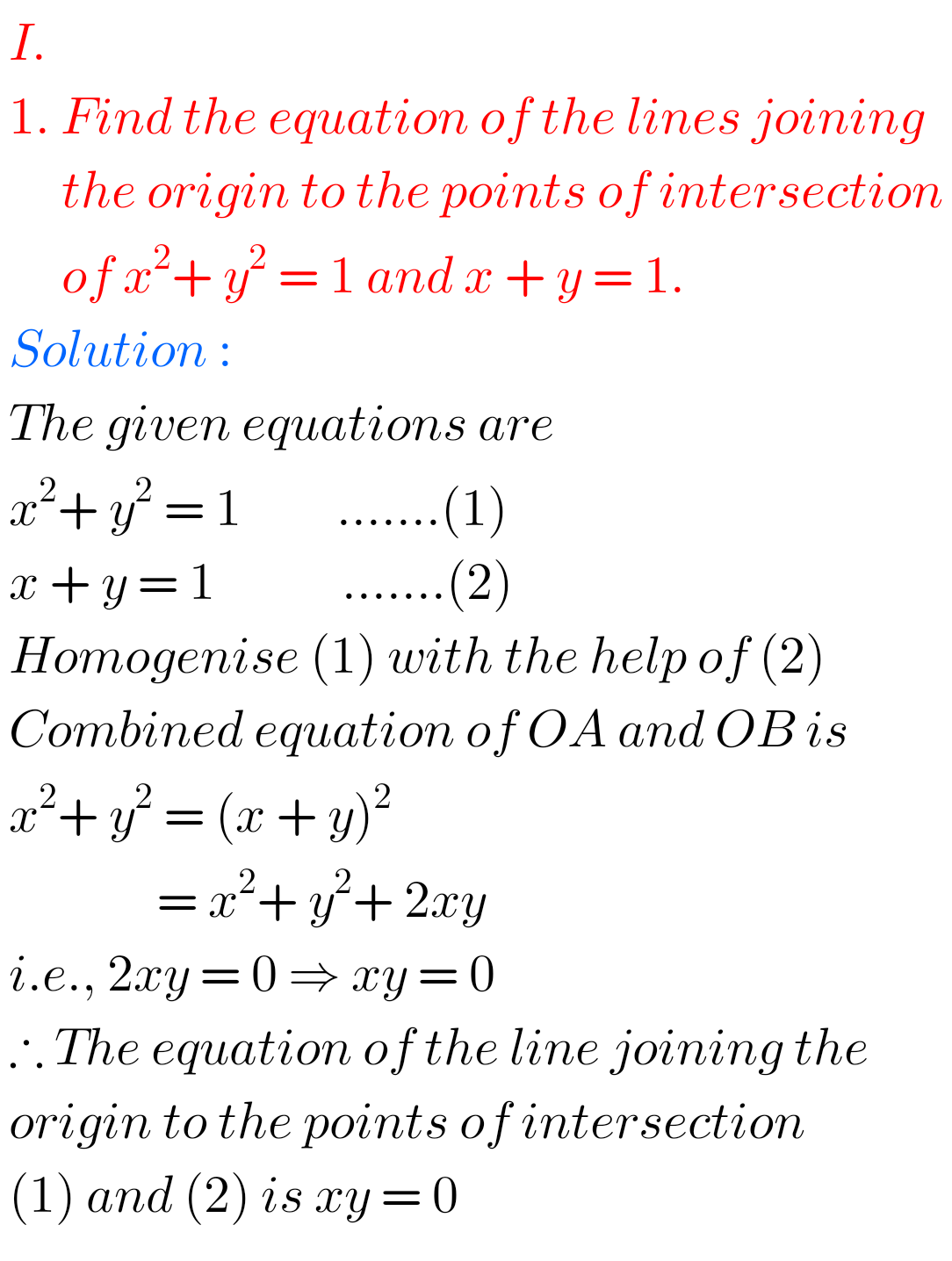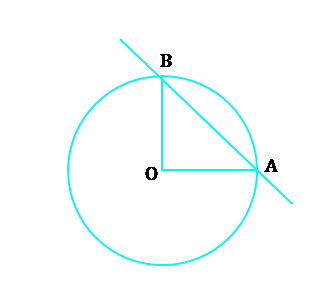## Maths Solutions for Pair of Straight Lines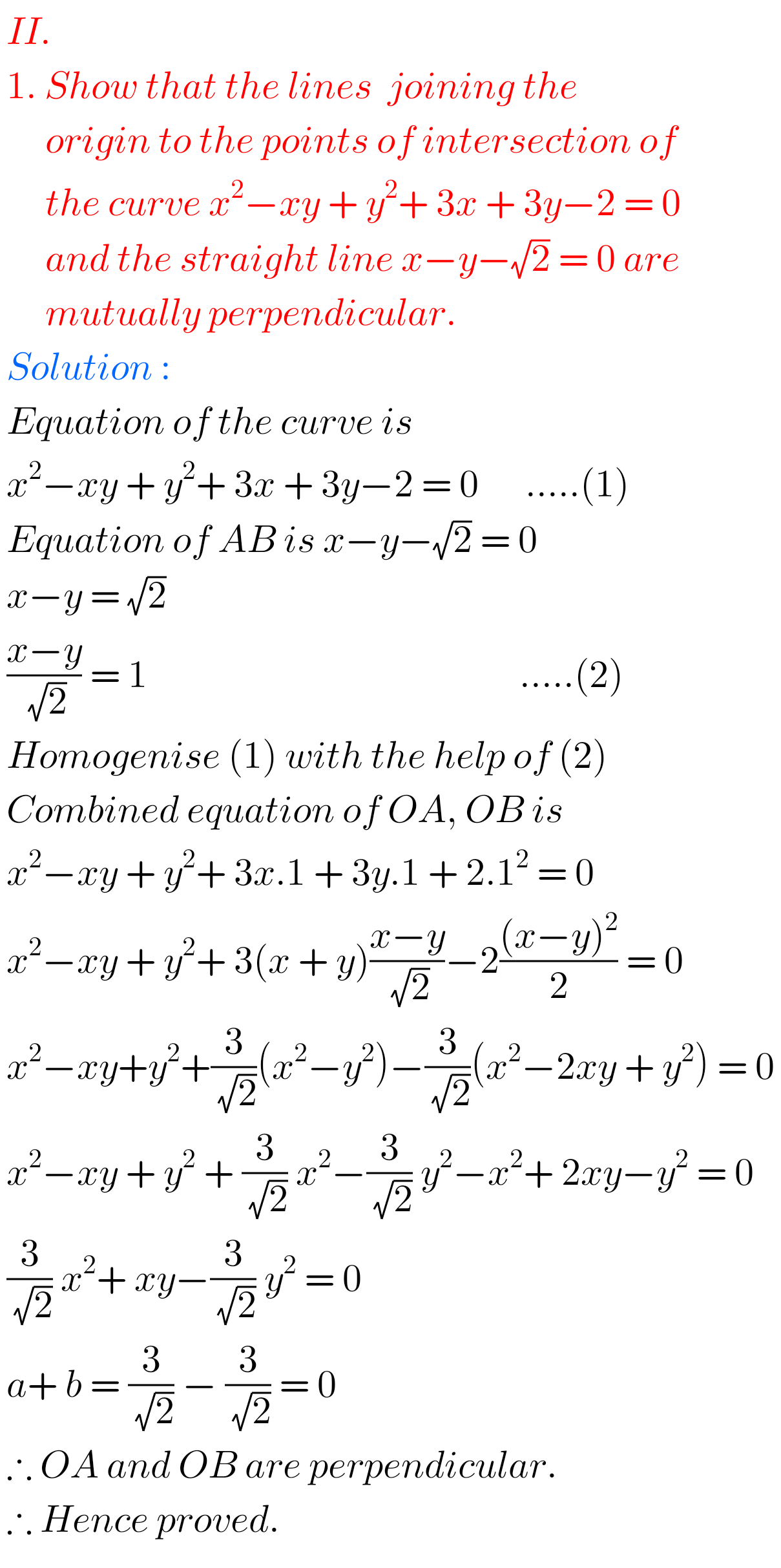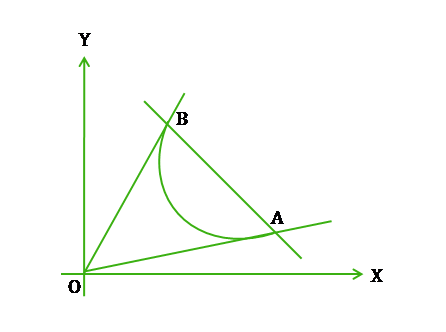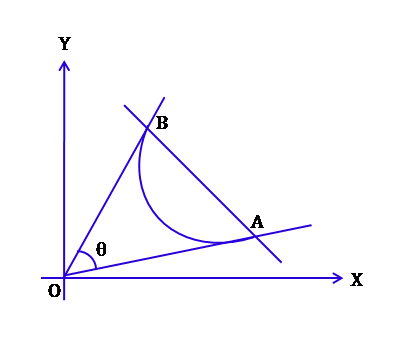## Inter Maths 1B Pair of Straight Lines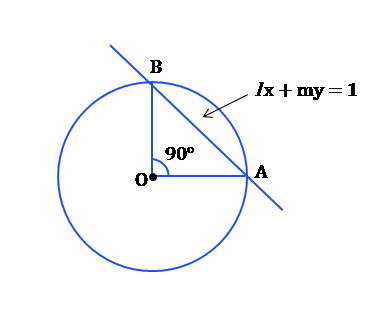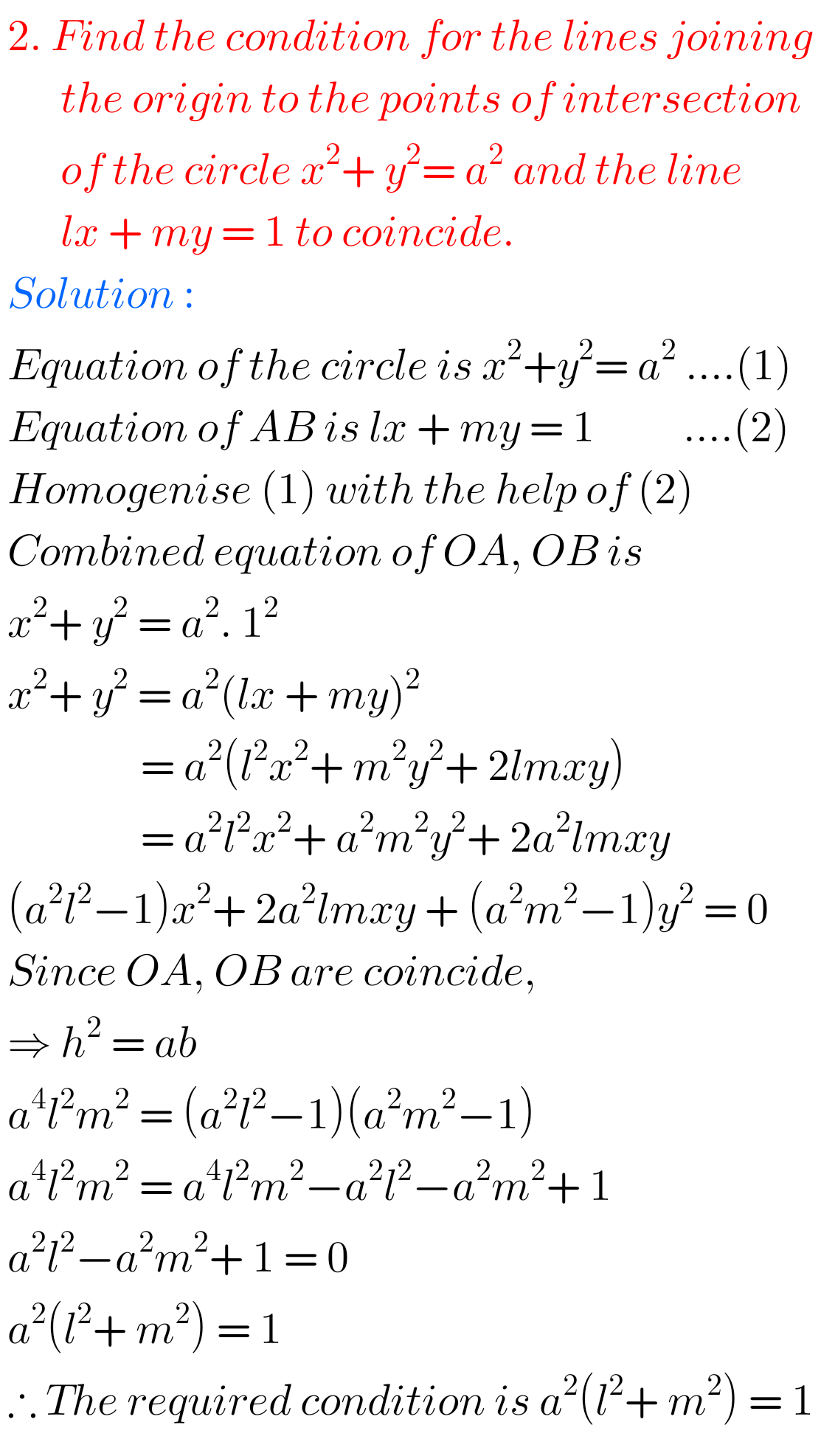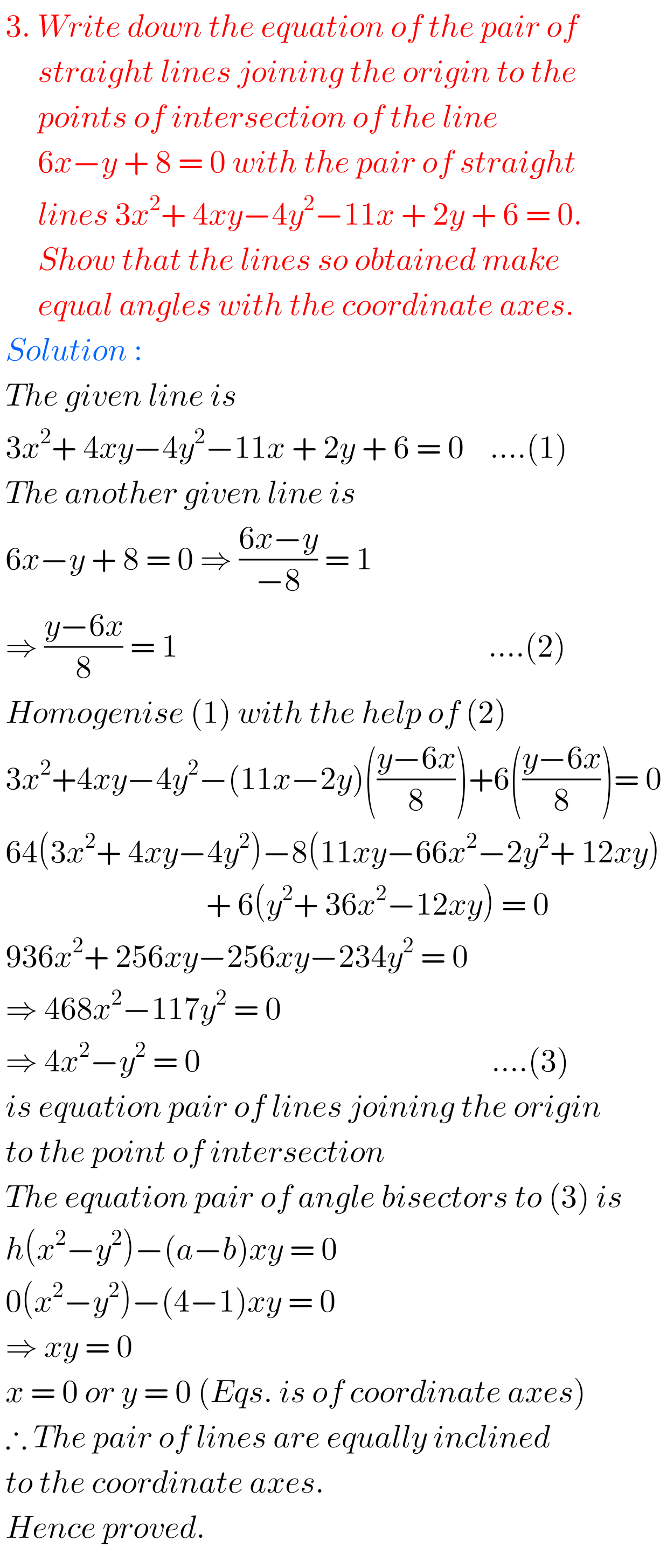Note : Observe the solutions and try them in your own method.You can also see the solutions for text book II A

Complex numbers solutions for textbook

De Moivre’s Theorem textbook solutions

Exercise 3(b)

Exercise 3(c)

You can also see solutions for Inter text book Maths IIB

1. Circle

3. Parabola

4. Ellipse